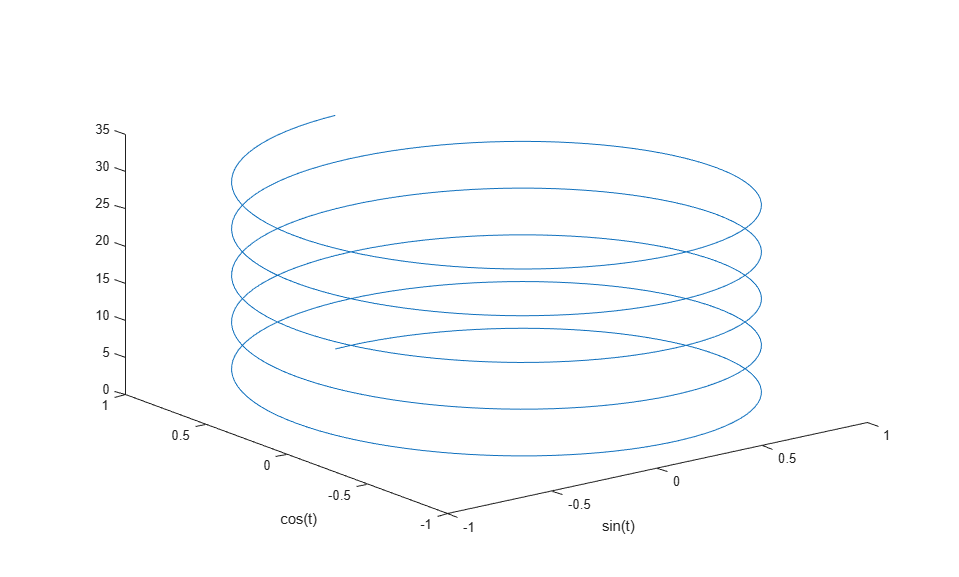## Creating 3-D Plots

This example shows how to create 3-D line plots in MATLAB using the plot3 function.

Create a regularly-spaced vector t from 0 to 10*pi using pi/50 as the increment between elements.

```t = 0:pi/50:10*pi; ```

Calculate sine for each value in t.

```st = sin(t); ```

Calculate cosine for each value in t.

```ct = cos(t); ```

Use the 'plot3' function to display a three-dimensional plot of a set of data points.

```figure; plot3(st, ct, t) xlabel('sin(t)') ylabel('cos(t)') ```